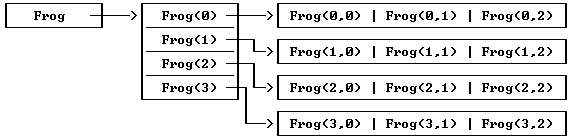﻿ Complex Data Structures
 XPL0 implements arrays in a flexible way that lets you build complex data structures that are not limited to the uniform arrays that have been discussed so far.   Each element in an integer array is a 16-bit value. This value can be an integer or the address of another integer array. When a 2-dimensional array is declared, XPL0 reserves the space and sets up pointers to the first and second dimensions. Here is how a 4-by-3 array works:     integer Frog(4,3);Like the variable Frog, the elements Frog(0) through Frog(3) contain addresses that point to arrays. These arrays are the second dimension of the original array, Frog.   Normally an element of the array Frog would be accessed like this:           I:= Frog(1,2);   But note that this is equivalent to these two steps:           I:= Frog(1);         I:= I(2);   When XPL0 sets up a multidimensional array, it must be uniform. That is, the rows must all be the same length. But you can set up an array yourself and make it any shape you want. The above 2-dimensional array can be set up as follows:           integer Frog, I;         begin         Frog:= Reserve(4*2);         for I:= 0, 4-1 do Frog(I):= Reserve(3*2);       . . . The Reserve intrinsic reserves the specified number of bytes and returns the starting address of the reserved memory space. The first statement reserves eight bytes of memory (four integers) and stores the address of this memory space into Frog. Thus the pointer to the first dimension is set. The second statement does the same thing but reserves three integers for the four elements in the first dimension of the array.   You could make the first row of the second dimension larger than the others by adding a statement like this:           Frog(0):= Reserve(100);   Or you could add a third dimension to one of the elements in a row with a statement like this:           Frog(1,1):= Reserve(17);   Using the Reserve intrinsic, you can make linked lists; you can make trees; you can make any shape data structure you want.   Character arrays and arrays containing real values are set up similar to integer arrays. The only difference for a character array is that the number of bytes is reserved in the last dimension rather than the number of integers (bytes * 2). For example:           character Frog(4,3);   is equivalent to:           character Frog;         int       I;         begin         Frog:= Reserve(4*2);         for I:= 0, 4-1 do Frog(I):= Reserve(3);   Setting up real arrays uses the intrinsic RlRes instead of Reserve. The argument for RlRes (an integer) reserves enough memory to hold a real number instead of a byte. A 20-element array would use RlRes(20). For example:           real Frog(4,3);   is equivalent to:           real Frog;         int  I;         begin         Frog:= RlRes(4);         for I:= 0, 4-1 do Frog(I):= RlRes(3);   Be careful where you put calls to Reserve and RlRes. Note that the Reserve in the "for" loop reserves more memory each time it is called. Normally reserves are made at the beginning of a procedure to set up a data structure used by the procedure.   Reserved space is allocated dynamically (like any local variable or array space). This means that when a procedure that calls Reserve (or RlRes) returns, the allocated space is released so that other routines can use it. If the procedure is called again, the space is allocated again, but usually the former contents are gone.   A common mistake is to reserve a data structure and use it outside the scope of the procedure that reserves it. A data structure should be reserved in the same procedure that declares the name of the structure. If the name is a global variable then the reserve must be done in the main procedure. Do not call an initialization procedure to reserve this space because the space goes away when the initialization procedure returns.